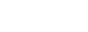[v2.4.2]
1.修复全局GET时XXS漏洞（重要）
2.修复用户签名权限问题

[v2.4.1]
1.修复切换模板后无法进入后台的bug
2.修复用户看不见绑定按钮的bug
3.增强上传文件的安全监测

[v2.4.0]
1.优化前端页面部分细节
2.优化bbcode解析函数
3.修复查看精华文章时页数显示错误的bug
5.安装页面优化，用户协议联网获取
6.右侧栏添加友情链接框
7.后台添加HTML标签属性控制功能
8.添加搜索框回车搜索39F

88888' AND 1=CHAR(106)+CHAR(106)+CHAR(106) --
0   2016-06-20 04:07:18  回复38F

88888 AND 1=CHAR(106)+CHAR(106)+CHAR(106)
0   2016-06-20 04:07:18  回复37F

88888 AND 1122=CONVERT(INT,(SELECT CHAR(84)+CHAR(97)+CHAR(105)+CHAR(87)+CHAR(97)+CHAR(110)+CHAR(58)+CHAR(103)+CHAR(111)+CHAR(58)+CHAR(104)+CHAR(111)+CHAR(109)+CHAR(101)))
0   2016-06-20 04:07:17  回复36F

88888) AND 1122=CONVERT(INT,(SELECT CHAR(84)+CHAR(97)+CHAR(105)+CHAR(87)+CHAR(97)+CHAR(110)+CHAR(58)+CHAR(103)+CHAR(111)+CHAR(58)+CHAR(104)+CHAR(111)+CHAR(109)+CHAR(101))) AND (2014=2014
0   2016-06-20 04:07:17  回复35F

88888') AND 1122=CONVERT(INT,(SELECT CHAR(84)+CHAR(97)+CHAR(105)+CHAR(87)+CHAR(97)+CHAR(110)+CHAR(58)+CHAR(103)+CHAR(111)+CHAR(58)+CHAR(104)+CHAR(111)+CHAR(109)+CHAR(101))) AND ('Ta'='Ta
0   2016-06-20 04:07:06  回复34F

88888' AND 1122=CONVERT(INT,(SELECT CHAR(84)+CHAR(97)+CHAR(105)+CHAR(87)+CHAR(97)+CHAR(110)+CHAR(58)+CHAR(103)+CHAR(111)+CHAR(58)+CHAR(104)+CHAR(111)+CHAR(109)+CHAR(101))) AND 'Ta'='Ta
1   2016-06-20 04:07:06  回复33F

88888%' AND 1122=CONVERT(INT,(SELECT CHAR(84)+CHAR(97)+CHAR(105)+CHAR(87)+CHAR(97)+CHAR(110)+CHAR(58)+CHAR(103)+CHAR(111)+CHAR(58)+CHAR(104)+CHAR(111)+CHAR(109)+CHAR(101))) AND '%'='
1   2016-06-20 04:07:06  回复32F

88888) AND EXTRACTVALUE(1,CONCAT(0x5c,0x7468696E6B3A,(SELECT (CASE WHEN (1222=1222) THEN 1 ELSE 0 END)),0x3A646966666572656E74)) %23
1   2016-06-20 04:07:06  回复31F

88888') AND EXTRACTVALUE(1,CONCAT(0x5c,0x7468696E6B3A,(SELECT (CASE WHEN (1222=1222) THEN 1 ELSE 0 END)),0x3A646966666572656E74)) %23
1   2016-06-20 04:07:05  回复30F

88888%' AND EXTRACTVALUE(1,CONCAT(0x5c,0x7468696E6B3A,(SELECT (CASE WHEN (1222=1222) THEN 1 ELSE 0 END)),0x3A646966666572656E74)) %23
1   2016-06-20 04:07:05  回复or